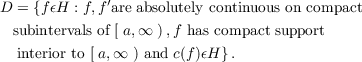#### Vol. 55, No. 1, 1974

 Download this articleFor screen For printingRecent Issues Vol. 325: 1  2 Vol. 324: 1  2 Vol. 323: 1  2 Vol. 322: 1  2 Vol. 321: 1  2 Vol. 320: 1  2 Vol. 319: 1  2 Vol. 318: 1  2Online Archive Volume: Issue:The Journal Subscriptions Editorial Board Officers Contacts Submission Guidelines Submission Form Policies for Authors ISSN: 1945-5844 (e-only) ISSN: 0030-8730 (print) Special Issues Author Index To Appear Other MSP Journals
Continuous spectra of a singular symmetric differential operator on a Hilbert space of vector-valued functions

### Robert Lee Anderson

Vol. 55 (1974), No. 1, 1–7
##### Abstract

Let H be the Hilbert space of complex vector-valued functions f :a,C2 such that f is Lebesgue measurable ona,and af(s)f(s)ds < . Consider the formally self adjoint expression c(y) = y′′ + Py ona,, where y is a 2-vector and P is a 2 × 2 symmetric matrix of continuous real valued functions ona,. Let D be the linear manifold in H defined byThen the operator L defined by L(y) = f(y),y𝜖D, is a real symmetric operator on D. Let L0 be the minimal closed extension of L. For this class of minimal closed symmetric operators this paper determines sufficient conditions for the continuous spectrum of self adjoint extensions to be the entire real axis. Since the domain, D0, of L0 is dense in H, self adjoint extensions of L0 do exist.

Primary: 47E05
##### Milestones